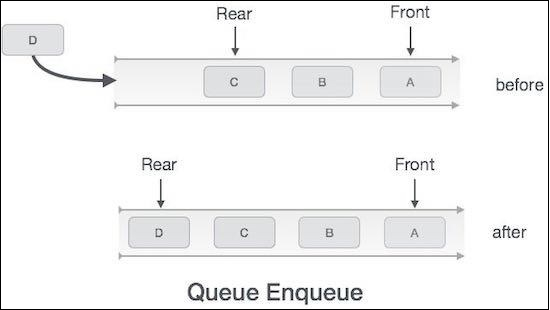# Add elements to a Queue using Javascript

Enqueuing elements to a Queue means adding them to the end of the array. We are taking the end of the container array to be the tail of the queue as we'll perform all insertions operations with respect to it.So we can implement the enqueue function as follows −

## Example

enqueue(element) {
// Check if Queue is full
if (this.isFull()) {
console.log("Queue Overflow!");
return;
}
// Since we want to add elements to end, we'll just push them.
.container.push(element);
}

You can check if this function is working fine using −

## Example

 let q = new Queue(2);
q.enqueue(1);
q.enqueue(2);
q.enqueue(3);
q.display();

## Output

This will give the output −

Queue Overflow!
[ 1, 2 ]